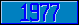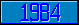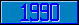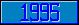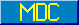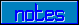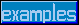## \$%MTXDET

### M[UMPS] by Example

```
2-Jul–96, 6:54:35

Routine Save for Matrix Mathematics Library function \$%MTXDET^MATH

;

; Unless otherwise noted, the code below

; was approved in document X11/96–26

;

; If corrections have been applied,

; first the original line appears,

; with three semicolons at the beginning of the line.

;

; Then the source of the correction is acknowledged,

; then the corrected line appears, followed by a

; line containing three semicolons.

;

MTXDET(A,N) ;

; Compute determinant of matrix A[N,N]

IF \$DATA(A)<10 QUIT ""

IF \$GET(N)<1 QUIT ""

;

; First the simple cases

;

IF N=1 QUIT \$GET(A(1,1))

IF N=2 QUIT \$GET(A(1,1))*\$GET(A(2,2))-(\$GET(A(1,2))*\$GET(A(2,1)))

;

NEW DET,I,SIGN

;

; Det A = sum (k=1:n) element (i,k) * cofactor [i,k]

;

SET DET=0,SIGN=1

FOR I=1:1:N DO

. SET DET=\$GET(A(1,I))*\$%MTXCOF^MATH(.A,1,I,N)*SIGN+DET

. SET SIGN=-SIGN

. QUIT

QUIT DET

;===

;

;

;

```